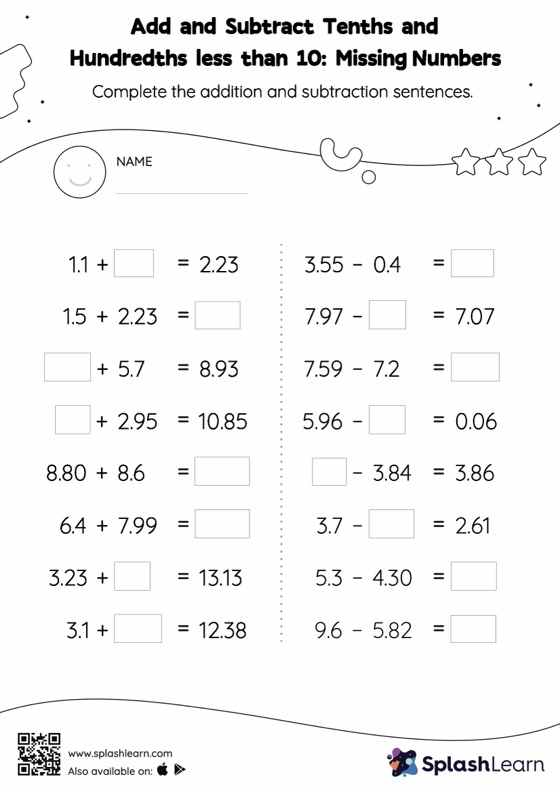# Add and Subtract Tenths and Hundredths less than 10: Missing Numbers Worksheet

Home > Add and Subtract Tenths and Hundredths less than 10: Missing NumbersGive your little one the opportunity to dive deeper into addition and subtraction with this add and subtract tenths and hundredths less than 10 worksheet. Students align the decimal points and use zero as space holders to add and subtract decimals. Then they use the relationship between addition and subtraction to find the missing number in add and subtract tenths and hundredths less than 10 worksheet. In each problem, the numbers are laid out in the horizontal format. Students should try to use different strategies involving composing and decomposing numbers to solve these problems. This will help them develop flexibility and fluency.